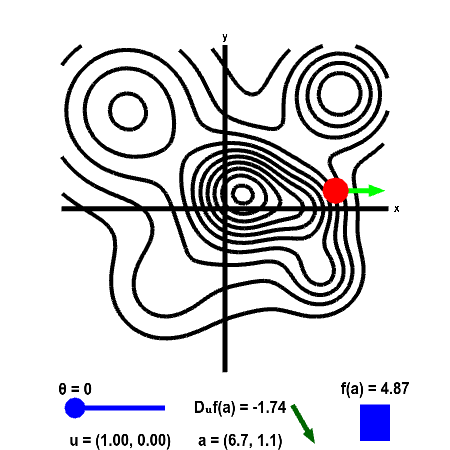# Math Insight

### Applet: Directional derivative on a mountain shown as level curvesThe height of a mountain range described by a function $f(x,y)$ is shown as a level curve plot. A point $\vc{a}$ (in red) can be moved with the mouse. The height $f(\vc{a})$ is shown by the bar in the lower right. The directional derivative is computed in the direction of the two-dimensional vector $\vc{u}$. This direction is illustrated by the light green vector, and the value of the direction $\vc{u}$ is shown in the lower left. The direction of $\vc{u}$ is determined by the angle $\theta$ it makes with straight east (positive $x$ direction). The angle $\theta$, and hence $\vc{u}$, can be changed using the slider. The value of the directional derivative $D_{\vc{u}}f(\vc{a})$ is shown at the bottom of the panel, along with the value of $\vc{a}$ itself. The value of $D_{\vc{u}}f(\vc{a})$ is the slope of the dark green vector to its right.

If you set $\vc{u}$ to point straight east ($\theta=0$ in the applet), then $\vc{u}$ points in the positive $x$ direction ($\vc{u}=(1,0)$) so that $\displaystyle D_{\vc{u}}f(\vc{a}) = \pdiff{f}{x}(\vc{a})$. Similarly, when $\vc{u}$ points straight north ($\theta=\pi/2$), then $\vc{u}$ points in the positive $y$ direction ($\vc{u}=(0,1)$) so that $\displaystyle D_{\vc{u}}f(\vc{a}) = \pdiff{f}{y}(\vc{a})$.

If you make $\vc{u}$ point in a direction parallel to the level curve, what happens to $D_{\vc{u}} f(\vc{a})$? (Since the height is constant along a level curve, you should be able to infer what the slope in that direction should be.) What happens to $D_{\vc{u}}f(\vc{a})$ when you turn $\vc{u}$ to point in the opposite direction (i.e., add or subtract $\pi$ from $\theta$)?